# Simultaneous Detection of Compromised Items and Examinees with Item Preknowledge using Response Time Information

Yes, you heard it right! This post introduces a model that uses response time information for simultaneous estimation of items being compromised and examinees having item preknowledge. The model improves upon the ideas laid out in Kasli et al. (2020), and further relaxes the assumption that the compromised items are known. The model is fitted using a Bayesian framework as implemented in Stan.

Cengiz Zopluoglu (University of Oregon)
6-24-2021

Acknowledgment. I want to thank Jacob Socolar and Luiz Max Carvalho from Stan Forums to give me a push in the right direction while working on this problem. The idea of marginalizing the discrete parameters in Stan was a difficult one to understand. This post was also handy if you are interested in the idea of marginalizing discrete parameters. This is another post that was very helpful to get an idea about how to fix some identification issues initially leading multi-modal posteriors.

Detecting item preknowledge is a complex problem. It is challenging to detect compromised items or fraudulent examinees at the same time. For instance, we don’t typically know who had item preknowledge, which is typically the purpose of analysis. We don’t necessarily know which items are compromised, although there may be specific scenarios that we know the set of compromised items. We don’t know whether the same examinees had access to the same set of items or different smaller subgroups of examinees had access to the different subsets of items. Maybe, there is some overlap among these compromised subsets used by different groups, maybe not. We don’t know if the examinees with item preknowledge had access to the items with the right keyed responses. So, they may respond faster but not necessarily correct to items they had seen before. We don’t know if the examinees manipulate their response time to obscure evidence to be used against them. So, they may intentionally spend longer times on items, but they give the correct response at the end to benefit from cheating. With many unknowns, researchers tend to simplify the problem by making assumptions and focusing on one aspect of the problem at a time. Some methods use either response time or item responses in their modeling. Some methods assume that the set of compromised items is known. Some methods assume that the group of examinees with item preknowledge is known. Some methods attempt to solve the problem in two stages or using iterative cycles, solving a smaller problem in each step/iteration.

In this post, I am playing with an improved version of Kasli et al. (2020). In the paper by Kasli et al. (2020), they described a model to detect examinees with item preknowledge by using response time data assuming that compromised items were known. This improved version of the model does not make that assumption. Instead, I also define item compromise status as a parameter, and estimate probabilities for items being compromised along with probabilities for examinees having item preknowledge at the same time. In a follow-up post, I will lay out the details of how item response data can also be incorporated into this model to provide more information in estimating these parameters.

## Lognormal Response Time Model

For those unfamiliar, van der Linden’s lognormal response time model (LNRT) is one of the available IRT models to model response time information. In this model, the observed response time for an examinee on an item is modeled through two item parameters and one person parameter. For example, suppose $$RT_{ij}$$ represents the log of the response time for the $$i^{th}$$ person on the $$j^{th}$$ item. There are two item parameters for each item, time intensity parameter ($$\beta_j$$) and time discrimination parameter ($$\alpha_j$$). There is also a latent speed parameter for each examinee ($$\tau_i$$).

The log of the response time for the $$i^{th}$$ person on the $$j^{th}$$ item is assumed to follow a normal distribution

$\begin{equation} RT_{ij} | \tau_{i},\alpha_j,\beta_j \sim N(\mu_{ij},\sigma_j) \tag{1} \end{equation}$

with a density function

$\begin{equation} f(RT_{ij} | \tau_{i},\alpha_j,\beta_j) = \frac{1}{\sigma_j \sqrt{2\pi}} e^{-\frac{1}{2}(\frac{RT_{ij} - \mu_{ij}}{\sigma_j})^2} \tag{2} \end{equation}$

where $$\mu_{ij}$$ and $$\sigma_j$$ are defined as

$\begin{equation} \mu_{ij} = \beta_j - \tau_{i} \tag{3} \end{equation}$

$\begin{equation} \sigma_{j} = \frac{1}{\alpha_j}. \tag{4} \end{equation}$

Below are some plots to get some insight into these parameters. The plots feature three different $$(\beta,\alpha)$$ combinations. The straight blue lines represent the expected response time, while the gray dashed lines represent the variability around the expected response time, 1.96 SD below and 1.96 SD above the expected response time.

• $$(\beta,\alpha) = (4,4)$$
• $$(\beta,\alpha) = (3,4)$$
• $$(\beta,\alpha) = (4,8)$$

In all these plots, the expected response time decreases as the latent speed increases. The LNRT model implies that an examinee with a higher latent speed is expected to respond faster to the administered items. The two plots in the first row present two hypothetical items with the same $$\alpha$$ parameter, but they differ in the $$\beta$$ parameters. If the same examinee responds to two items, the observed response time is expected to be smaller for the item with the lower $$\beta$$ parameter. $$\beta$$ parameter represents how much time an item require to respond for an average examinee. LNRT implies that items with smaller time-intensity parameters require less time to respond than items with higher time-intensity parameters. The two plots in the second row present two hypothetical items with the same $$\beta$$ parameter, but they differ in the $$\alpha$$ parameters. Notice that everything else being equal, there is more variance in response time when for an item with a lower $$\alpha$$. LNRT implies that the items with lower time-discrimination parameters have more noise and contributing factors to response time other than an examinee’s latent speed.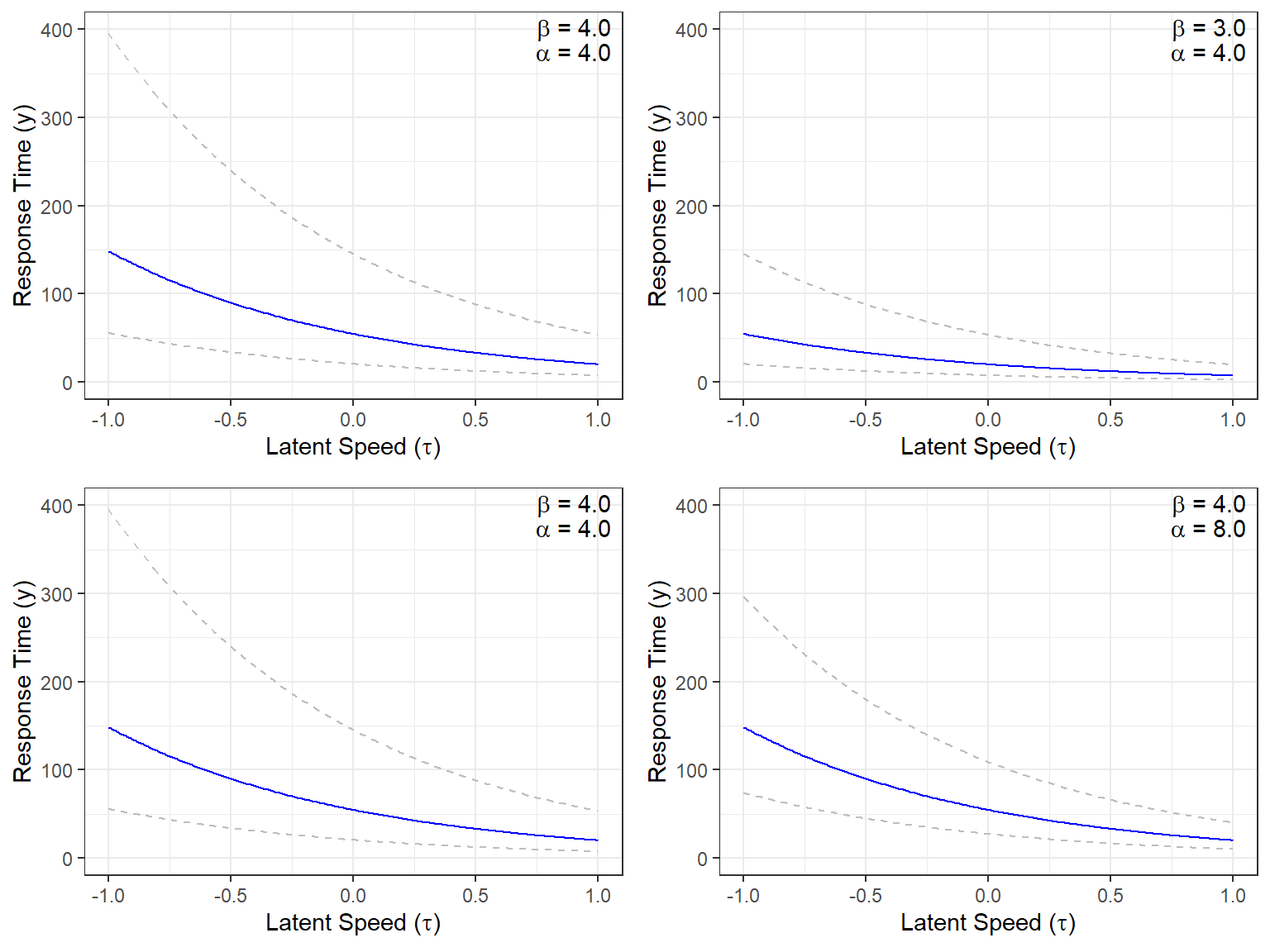## Let’s make the model a little bit more complex!

We will add a few more parameters to the original LNRT model to detect examinees with item preknowledge and items being compromised simultaneously. These modifications are inspired by the Deterministic Gated IRT model by Shu et al. (2013). In an earlier attempt by Kasli et al. (2020), we applied the idea of Shu et al. (2013) to modeling response times. However, a critical limitation for both Shu et al. (2013) and Kasli et al. (2020) is that they assume the set of compromised items is known, and this information enters into the model as data. In this post, I try to improve it further by relaxing that assumption. Instead of assuming that the compromised status of each item is known, I estimate this as a parameter.

I first hypothesize that there are two latent speed parameters for each examinee, a true latent speed parameter and a cheating latent speed parameter. The model operationalizes the true latent speed when responding to an uncompromised item ($$\tau_{ti}$$) and the cheating latent speed when responding to a compromised item ($$\tau_{ci}$$). In addition, we add a discrete person parameter for each examinee ($$H_i$$) indicating whether an examinee has item preknowledge (0: examinee does not have preknowlegde, 1: examinee has preknowledge) and add a discrete parameter for each item ($$C_j$$) indicating whether or not an item is compromised.

In this modified model, the log of the response time the $$i^{th}$$ person on the $$j^{th}$$ item is assumed to follow a normal distribution

$\begin{equation} RT_{ij} | \tau_{ti},\tau_{ci},H_i,\alpha_j,\beta_j,C_j \sim N(\mu_{ij},\sigma_j) \tag{5} \end{equation}$

with a density function

$\begin{equation} f(RT_{ij} | \tau_{ti},\tau_{ci},H_i,\alpha_j,\beta_j,C_j) = \frac{1}{\sigma_j \sqrt{2\pi}} e^{-\frac{1}{2}(\frac{RT_{ij} - \mu_{ij}}{\sigma_j})^2} \tag{6} \end{equation}$

where $$\mu_{ij}$$ and $$\sigma_j$$ are defined as

$\begin{equation} \mu_{ij} = (\beta_j - \tau_{ti})^{1-H_i} \times \Big (C_j \times (\beta_j - \tau_{ci}) + (1-C_j) \times (\beta_j - \tau_{ti} \Big )^{H_i} \tag{7} \end{equation}$

$\begin{equation} \sigma_{j} = \frac{1}{\alpha_j}. \tag{8} \end{equation}$

Eq. (7) seems a bit confusing. It just indicates that the expected response time is equal to $\beta_j - \tau_{ci},$

when an examinee has item preknowledge and responds to a compromised item ($$H_i=1, C_j=1$$), and equal to

$\beta_j - \tau_{ti},$

in all other three scenarios,($$H_i=1,C_j=0$$; $$H_i=0,C_j=1$$; $$H_i=0,C_j=0$$).

To implement the model in Stan, we also have to rewrite the original density function to marginalize the discrete parameters. Stan, in its current form, cannot handle discrete parameters in the model. Therefore, we have to explicitly write the original density function for every possible combination of the discrete parameters. Later, we will use the following to model the response time.

\begin{aligned} f(RT_{ij}| \tau_{ti},\tau_{ci},H_i,\alpha_j,b_j,C_j) = f(RT_{ij}| \tau_{ti},\tau_{ci},\alpha_j,\beta_j,C_j=1,H_i=1) \times P(C_j = 1) \times P(H_i =1) +\\ f(RT_{ij}| \tau_{ti},\tau_{ci},\alpha_j,\beta_j,C_j=1,H_i=0) \times P(C_j = 1) \times P(H_i =0) +\\ f(RT_{ij}| \tau_{ti},\tau_{ci},\alpha_j,\beta_j,C_j=0,H_i=1) \times P(C_j = 0) \times P(H_i =1) +\\ f(RT_{ij}| \tau_{ti},\tau_{ci},\alpha_j,\beta_j,C_j=0,H_i=0) \times P(C_j = 0) \times P(H_i =0) \end{aligned} Or, we can write it in a less cluttered way.

\begin{aligned} f(RT_{ij}| \tau_{ti},\tau_{ci},H_i,\alpha_j,\beta_j,C_j) = f(RT_{ij}| \tau_{ci},\alpha_j,\beta_j) \times P(C_j = 1) \times P(H_i =1) +\\ f(RT_{ij}| \tau_{ti},\alpha_j,\beta_j) \times P(C_j = 1) \times P(H_i =0) +\\ f(RT_{ij}| \tau_{ti},\alpha_j,\beta_j) \times P(C_j = 0) \times P(H_i =1) +\\ f(RT_{ij}| \tau_{ti},\alpha_j,\beta_j) \times P(C_j = 0) \times P(H_i =0) \end{aligned}

$$P(C_j = 1)$$ represents the probability of the jth item being compromised and $$P(H_i = 1)$$ represents the probability of the ith examinee having item preknowledge. Notice that we consider four possible combinations of the discrete parameters and write the density for each possible combination. While the density relies on $$\tau_{ci}$$ when ($$H_i=1, C_j=1$$), it relied on $$\tau_{ti}$$ for other three possible combinations.

## Model Identification and Prior Specifications

We assume that the joint distribution of true latent speed and cheating latent speed follow a multivariate normal distribution.

$\begin{pmatrix} \tau_{t}\\ \tau_{c} \end{pmatrix} = N(\mu_{\mathcal{P}},\Sigma_{\mathcal{P}} )$

with $$\mu_{\mathcal{P}}$$ is a vector of means and $$\Sigma_{\mathcal{P}}$$ is the covariance matrix decomposed into a diagonal matrix of standard deviations and a correlation matrix for person parameters.

$\Sigma_{\mathcal{P}} = \begin{pmatrix} \sigma_{\tau_t} & 0\\ 0 & \sigma_{\tau_c} \end{pmatrix} \Omega_\mathcal{P} \begin{pmatrix} \sigma_{\tau_t} & 0\\ 0 & \sigma_{\tau_c} \end{pmatrix},$

$\Omega_\mathcal{P}= \begin{pmatrix} 1 & \rho_{\tau_t,\tau_c}\\ \rho_{\tau_c,\tau_t} & 1 \end{pmatrix}$

This decomposition is a recommended practice in Stan User’s Guide. For model identification purposes, the mean vector of person parameters are fixed to zero, $\mu_{\mathcal{P}} = (0,0).$ The standard deviations and the correlation matrix are parameters to be estimated with the following priors:

$\sigma_{\tau_t} \sim exp(1) \\ \sigma_{\tau_c} \sim exp(1) \\ \Omega_{\mathcal{P}} \sim LKJ(1)$

Lewandowski-Kurowicka-Joe (LKJ) distribution with a parameter 1 is used as a prior for the correlation matrices as recommended in the Stan User’s Guide. For more information about the LKJ distribution, also see this link.

The item parameters are similarly assumed to follow a multivariate normal distribution. The only caveat is that I prefer working with the log of the $$\alpha$$ parameter.

$\begin{pmatrix} ln(\alpha) \\ \beta \end{pmatrix} = N(\mu_{\mathcal{I}},\Sigma_{\mathcal{I}} )$

with $$\mu_{\mathcal{I}}$$ is a vector of means and $$\Sigma_{\mathcal{I}}$$ is the covariance matrix decomposed into a diagonal matrix of standard deviations and a correlation matrix for item parameters.

$\Sigma_{\mathcal{I}} = \begin{pmatrix} \sigma_{ln(\alpha)} & 0 \\ 0 & \sigma_{\beta} \end{pmatrix} \Omega_\mathcal{I} \begin{pmatrix} \sigma_{ln(\alpha)} & 0\\ 0 & \sigma_{\beta} \end{pmatrix}$

$\Omega_\mathcal{I}=\begin{pmatrix} 1 & \rho_{ln(\alpha),\beta}\\ \rho_{ln(\alpha),\beta} & 1 \end{pmatrix}$

The following priors can be used for the parameters related to item characteristics.

$\mu_{ln(\alpha)} \sim N(0,0.5) \\ \sigma_{ln(\alpha)} \sim exp(1) \\ \mu_{\beta} \sim N(4,1) \\ \sigma_{\beta} \sim exp(1) \\ \Omega_{\mathcal{I}} \sim LKJ(1)$

Finally, we can define the following non-informative priors on the probability that a person is having item preknowledge and the probability that an item is being compromised.

$P(H_i =1) \sim Beta(1,1) \\ P(C_j =1) \sim Beta(1,1)$

## Stan Model Syntax

I will try to explain below how we can fit the described model with these specifications in Stan.

First, the data block provides the input data. I only specify the number of examinees (I), the number of items (J), and the I x J matrix, including the log of response time for each examinee on each item.

data{
int <lower=1> I;                       // number of examinees
int <lower=1> J;                       // number of items
real RT[I,J];                          // matrix  the log of responses
}

Then, we define the model parameters in the parameters block. In this block, we define every single parameter describe earlier in the model, $$\mu_{ln(\alpha)}$$, $$\sigma_{ln(\alpha)}$$, $$\mu_{\beta}$$, $$\sigma_{\beta}$$, $$\sigma_{\tau_t}$$, $$\sigma_{\tau_c}$$, an array for individual $$\tau_t$$ and $$\tau_c$$ parameters, an array for item parameters, $$\Omega_P$$, $$\Omega_I$$, $$P(C)$$, and $$P(H)$$.

parameters {
real mu_beta;                 // mean for time intensity parameters
real<lower=0> sigma_beta;     // sd for time intensity parameters

real mu_alpha;                // mean for log of time discrimination parameters
real<lower=0> sigma_alpha;    // sd for time discrimination parameters

real<lower=0> sigma_taut;     // sd for tau_t
real<lower=0> sigma_tauc;     // sd for tau_c

corr_matrix omega_P;       // 2 x 2 correlation matrix for person parameters
corr_matrix omega_I;       // 2 x 2 correlation matrix for item parameters

vector<lower=0,upper=1>[J] pC; // vector of length J for the probability of item compromise status

vector<lower=0,upper=1>[I] pH; // vector of length I for the probability of examinee item peknowledge

ordered person[I];           // an array with length I for person specific latent parameters
// Each array has two elements
// first element is tau_t
// second element is tau_c
// ordered vector assures that tau_c > tau_t for every person
// to make sure chains are exploring the same mode and
// multiple chains do not go east and west leading multi-modal posteriors

vector item[J];           // an array with length J for item specific parameters
// each array has two elements
// first element is alpha
// second element is beta
}
}

In the parameters block, notice that I use ordered person[I] when I define the array for person parameters instead of just writing vector person[I]. This is something you can do in Stan if you want to force an order for the vector elements. In this case, this is forcing $$\tau_c$$ to be larger than $$\tau_t$$ for every single person. It appeared that this was really important; otherwise, you would get multi-modal posterior distributions due to the different chains going to different directions for P(C) and P(H) parameters. For instance, see below an example for a parameter before I fixed this problem. It took a while to understand the importance of it and to figure out how to fix the problem. Once I forced $$\tau_c$$ to be larger than $$\tau_t$$ by using ordered instead of plain vector, it resolved the direction issue for these parameters.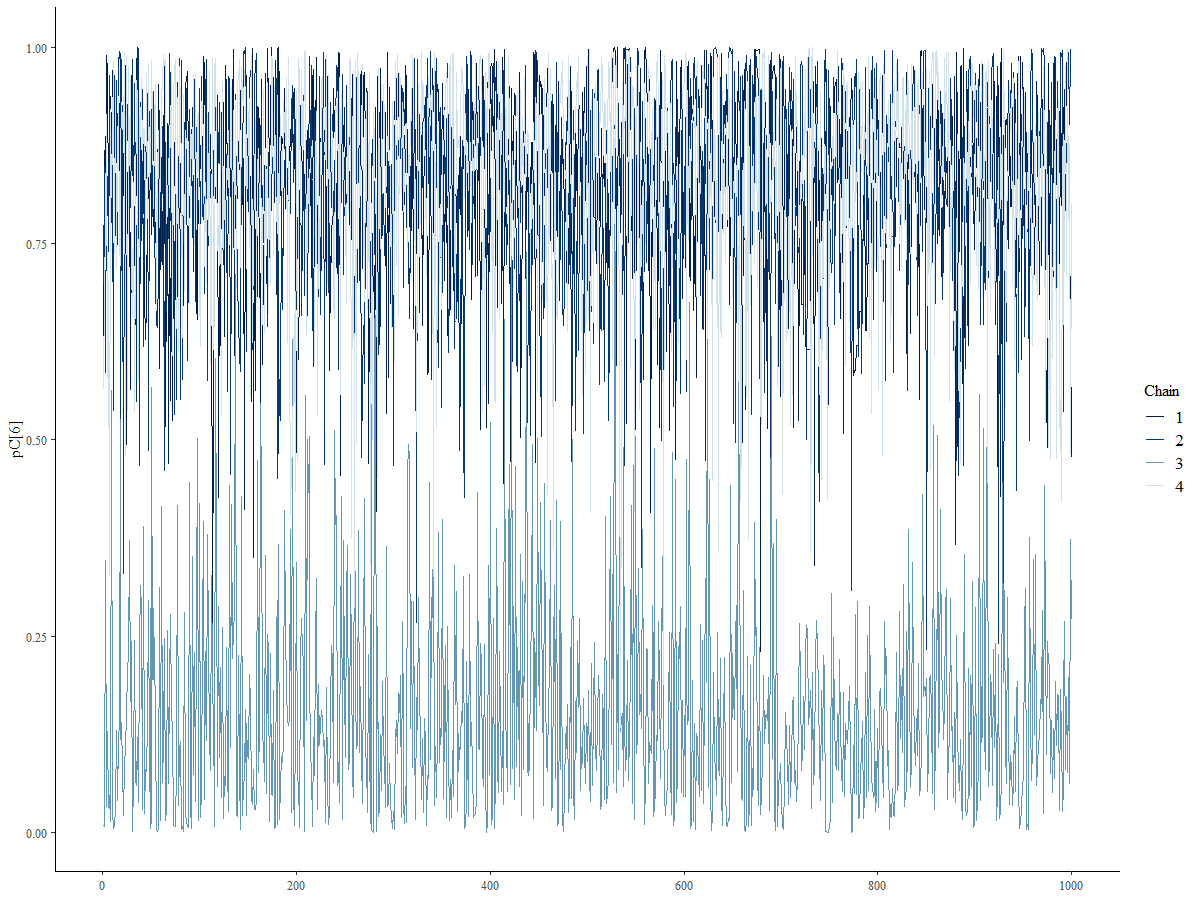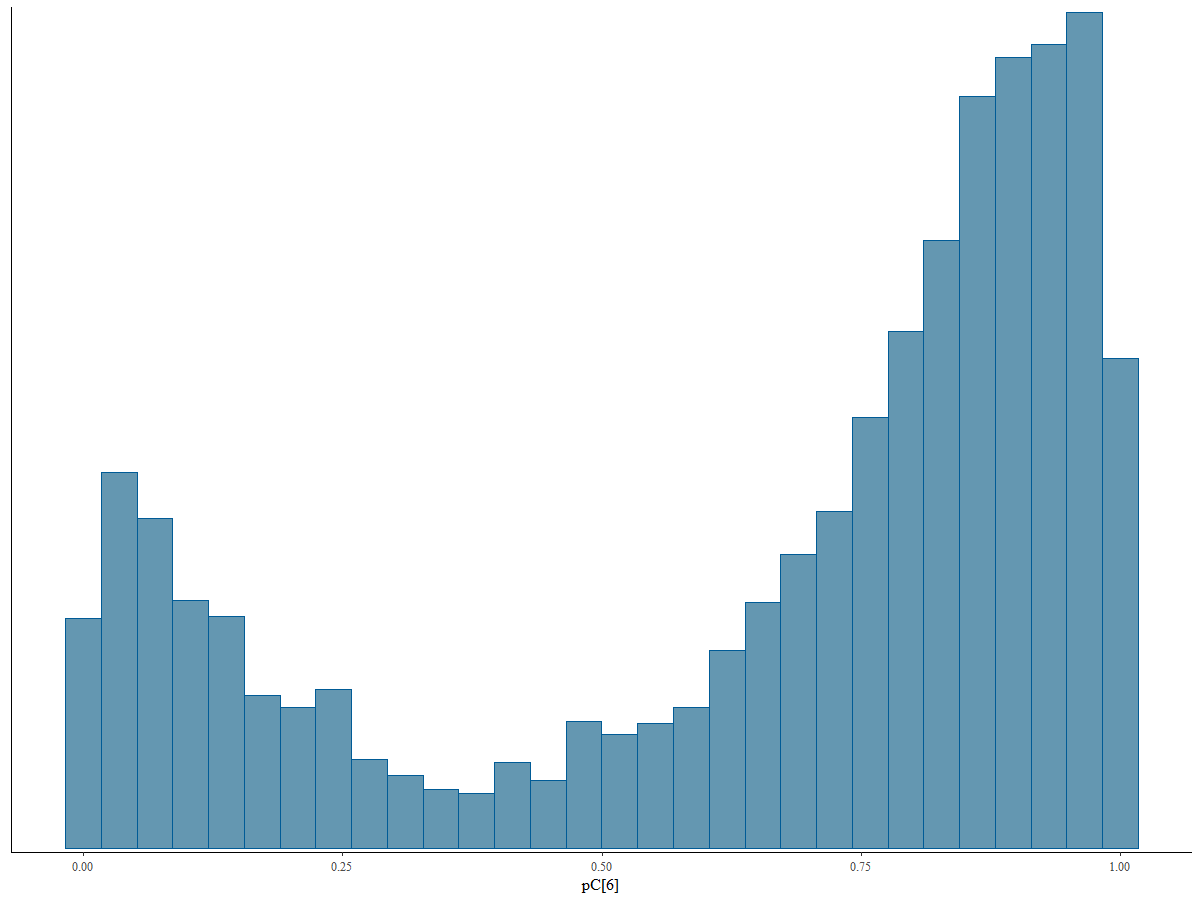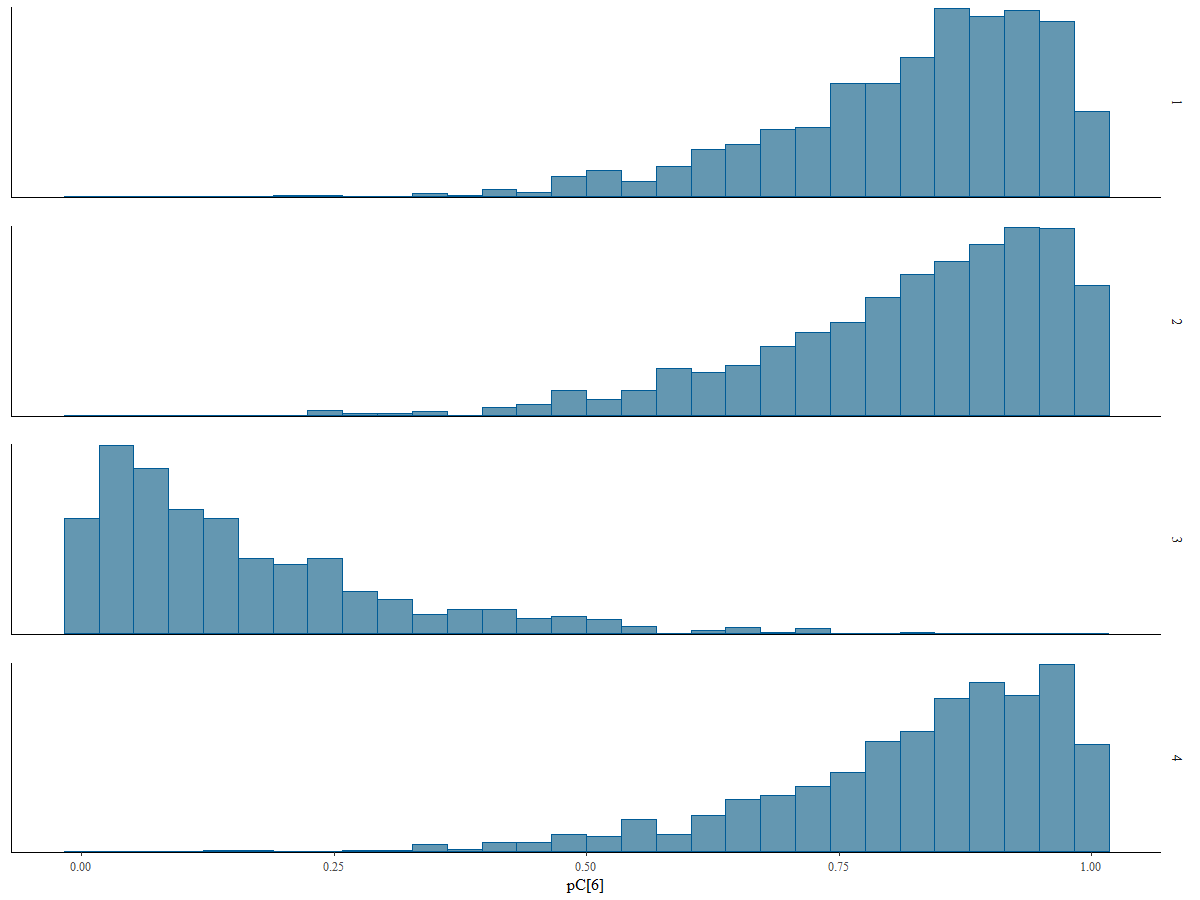We will need to have a transformed parameters block. In this block, we define the vector of means and vector of standard deviations for item and person parameters later to be used in the model block. As we draw the person and item parameters from a multivariate normal distribution, the parameters defined in the parameters block are combined into vector forms in the transformed parameters block. For instance,

• $$\mu_{ln(\alpha)}$$ and $$\mu_{\beta}$$ becomes $$\mu_\mathcal{I} = (\mu_{ln(\alpha)},\mu_{\beta})$$;

• a vector of fixed means for person parameters is formed as $$\mu_\mathcal{P} = (0,0)$$;

• $$\sigma_{\alpha}$$,$$\sigma_{\beta}$$, and $$\Omega_\mathcal{I}$$ are used to form the item parameter covariance matrix ($$\Sigma_\mathcal{I}$$) through quad_form_diag function in Stan; and,

• $$\sigma_{\tau_t}$$,$$\sigma_{\tau_c}$$, and $$\Omega_\mathcal{P}$$ are used to form the person parameter covariance matrix ($$\Sigma_\mathcal{I}$$) through quad_form_diag function in Stan.

transformed parameters{

vector mu_P;                        // vector for mean vector of person parameters
vector mu_I;                        // vector for mean vector of item parameters

vector scale_P;                     // vector of standard deviations for person parameters
vector scale_I;                     // vector of standard deviations for item parameters

cov_matrix Sigma_P;                 // covariance matrix for person parameters
cov_matrix Sigma_I;                 // covariance matrix for person parameters

mu_P = 0;
mu_P = 0;

scale_P = sigma_taut;
scale_P = sigma_tauc;

mu_I = mu_alpha;
mu_I = mu_beta;

scale_I = sigma_alpha;
scale_I = sigma_beta;

}

Finally, we specify the distributions and model in the model block.

model{

sigma_taut  ~ exponential(1);
sigma_tauc  ~ exponential(1);
sigma_beta  ~ exponential(1);
sigma_alpha ~ exponential(1);

mu_beta      ~ normal(4,1);
mu_alpha     ~ lognormal(0,0.5);

pC ~ beta(1,1);
pH ~ beta(1,1);

omega_P   ~ lkj_corr(1);
omega_I   ~ lkj_corr(1);

person  ~ multi_normal(mu_P,Sigma_P);

item    ~ multi_normal(mu_I,Sigma_I);

for (i in 1:I) {
for(j in 1:J) {

// item[j,1] represents log of parameter alpha of the jth item
// that's why we use exp(item[j,1]) below
// item[j,2] represents parameter beta of the jth item

//person[i,1] represents parameter tau_t of the ith person
//person[i,2] represents parameter tau_c of the ith person

real p_t = item[j,2]-person[i,1];   //expected response time for non-cheating response
real p_c = item[j,2]-person[i,2];  //expected response time for cheating response

// log of probability densities for each combination of two discrete parameters
// (C,T) = {(0,0),(0,1),(1,0),(1,1)}

real lprt1 = log1m(pC[j]) + log1m(pH[i]) + normal_lpdf(RT[i,j] | p_t, 1/exp(item[j,1]));  // T = 0, C=0
real lprt2 = log1m(pC[j]) + log(pH[i])   + normal_lpdf(RT[i,j] | p_t, 1/exp(item[j,1]));  // T = 1, C=0
real lprt3 = log(pC[j])   + log1m(pH[i]) + normal_lpdf(RT[i,j] | p_t, 1/exp(item[j,1]));  // T = 0, C=1
real lprt4 = log(pC[j])   + log(pH[i])   + normal_lpdf(RT[i,j] | p_c, 1/exp(item[j,1]));  // T = 1, C=1

target += log_sum_exp([lprt1, lprt2, lprt3, lprt4]);

}
}

}

The whole Stan syntax for the model can be saved as a stan file (Download the Stan model syntax).

To test if the model can successfully be fitted and how well it works, I will first test it using a simulated data and then using the experimental data from Toton and Maynes (2019).

## Simulated Data Example

### Data Generation

I will first simulate a dataset to test the performance the model. The followings are some important variables to consider in this simulation:

• There are 200 hypothetical examinees responding to 30 items.
• 40 hypothetical examinees had prior knowledge for 15 items.
• $$\beta$$ parameters are normally distributed with a mean of 4 and standard deviation of 0.5.
• $$\alpha$$ parameters are normally distributed with a mean of 2 and standard deviation of 0.5.
• $$\tau_t$$ and $$\tau_c$$ parameters follow a multivariate normal distribution with the following parameters:

$\begin{pmatrix} \tau_t \\ \tau_c \end{pmatrix} = N(\mu_{\mathcal{P}},\Sigma_{\mathcal{P}} )$

with $$\mu_{\mathcal{P}} = (0,0.4)$$ and

$\Sigma_{\mathcal{P}} = \begin{pmatrix} 0.0100 \ \ 0.0105 \\ 0.0105 \ \ 0.0225 \end{pmatrix}.$

The specifications about $$\tau_t$$ and $$\tau_c$$ correspond to a correlation of 0.7 between true latent speed and cheating latent speed, an about %35 reduction in response time on average when an examinees responds to a compromised item. Below are the code to simulate response time data with these specifications.

require(MASS)

set.seed(06202021)

N = 200    # number of examinees
n = 30     # number of items

# Time intensity parameters

beta  <- rnorm(n,4,.5)

# Time discrimination parameters

alpha <- rnorm(n,2,0.5)

# Tau_t and tau_c

tau <- mvrnorm(N,
mu = c(0,0.4),
Sigma = matrix(c(0.01,0.0105,0.0105,0.0225),2,2))

tau_t <- tau[,1]
tau_c <- tau[,2]

# Randomly select (approximately) 20% of examinees as having item prekowledge

H <- rbinom(N,1,.2)

# Randomly select (approximately) 50% of items as compromised

C <- rbinom(n,1,.5)

# Generate observed response times according to the model

rt <- matrix(nrow=N,ncol=n)

for(i in 1:N){
for(j in 1:n){

p_t <- beta[j] - tau_t[i]
p_c <- beta[j] - tau_c[i]

if(H[i] == 1 & C[j] == 1){
rt[i,j] = exp(rnorm(1,p_c,1/alpha[j]))
} else {
rt[i,j] = exp(rnorm(1,p_t,1/alpha[j]))
}

}
}

# Convert it to data frame and add group membership and a unique ID

rt       <- as.data.frame(rt)
rt$group <- H rt$id    <- 1:nrow(rt)

# Check the data


      V1     V2     V3     V4    V5    V6     V7     V8     V9    V10
1 128.22  47.50  65.83  82.58 17.13 35.31  24.21 184.04  29.48 14.449
2 353.25 126.99 191.42 110.42 53.50 76.33  33.65  95.92 163.65 13.782
3  72.86  47.72 116.38 157.30 86.60 28.66 123.08  52.51  67.22 22.403
4  91.98  51.01 137.09 167.42 30.12 95.76  40.70 219.40  33.01 75.115
5  25.87  78.50  76.75 175.06 68.95 33.80  30.32  59.24  72.79  4.150
6 256.00 107.92  73.04  71.10 82.48 38.20  68.92  34.24  41.14  2.515
V11   V12   V13    V14   V15    V16   V17   V18   V19   V20
1 37.07 29.56 51.73 106.69 39.70  49.75 62.55 44.58 58.64 107.4
2 38.90 13.66 79.53  91.17 49.38  67.88 65.12 70.00 47.08  17.3
3 40.04 18.19 61.68  72.10 44.50 223.67 43.79 49.39 44.58 235.2
4 50.57 43.59 49.65  96.93 86.55  55.34 41.29 32.95 42.70 329.3
5 70.53 24.22 21.34  63.83 43.70 157.27 82.45 35.00 75.03 147.9
6 64.42 20.53 41.58  79.14 45.88  45.94 50.70 21.43 32.15 266.0
V21    V22   V23     V24    V25   V26   V27    V28   V29   V30
1  79.04 100.73 103.3   6.736 104.52 32.80 87.32  61.66 61.07 43.29
2 187.34 196.59 176.8  86.660  47.96 40.85 83.93  78.75 59.47 12.33
3  85.58  48.59 136.2  79.418 101.56 40.03 65.18  24.61 67.75 41.38
4  62.51  73.77 118.6 225.904 158.70 29.83 42.67  48.28 56.17 48.89
5 144.93  52.72 180.7 997.649  66.67 32.88 42.35 121.88 61.07 20.01
6  59.06  54.31  68.0 190.259  53.75 20.64 40.75  31.81 75.62 16.28
group id
1     0  1
2     0  2
3     0  3
4     0  4
5     0  5
6     1  6
  # Reshape it to long format (for plotting purposes)

rt.long <- reshape(data        = rt,
idvar       = 'id',
varying     = list(colnames(rt)[1:n]),
timevar     = "Item",
times       = 1:n,
v.names      = "RT",
direction   = "long")

rt.long$compromised <- NA for(j in 1:n){ rt.long[rt.long$Item==j,]$compromised = C[j] } head(rt.long)   group id Item RT compromised 1.1 0 1 1 128.22 0 2.1 0 2 1 353.25 0 3.1 0 3 1 72.86 0 4.1 0 4 1 91.98 0 5.1 0 5 1 25.87 0 6.1 1 6 1 256.00 0 ### Data Check Below boxplots provide a snapshot of distributions for the generated response times for hypothetetical examinees with and without item preknowledge for compromised and uncompromised items. Not surprisingly, average log response time is about same for two groups of examinees for uncompromised items while examinees with item preknowledge respond slightly faster to compromised items. require(ggplot2) require(gridExtra) p1 <- ggplot(rt.long[rt.long$compromised==0,],
aes(x=factor(Item), y=log(RT),fill=factor(group))) +
geom_boxplot()+
theme_bw() +
xlab('Item Number')+
ylab('Log of Response Time')+
guides(fill=guide_legend(title="Group"))+
ggtitle('Uncompromised Items')

p2 <- ggplot(rt.long[rt.long$compromised==1,], aes(x=factor(Item), y=log(RT),fill=factor(group))) + geom_boxplot()+ theme_bw() + xlab('Item Number')+ ylab('Log of Response Time')+ guides(fill=guide_legend(title="Group"))+ ggtitle('Compromised Items') grid.arrange(p1,p2)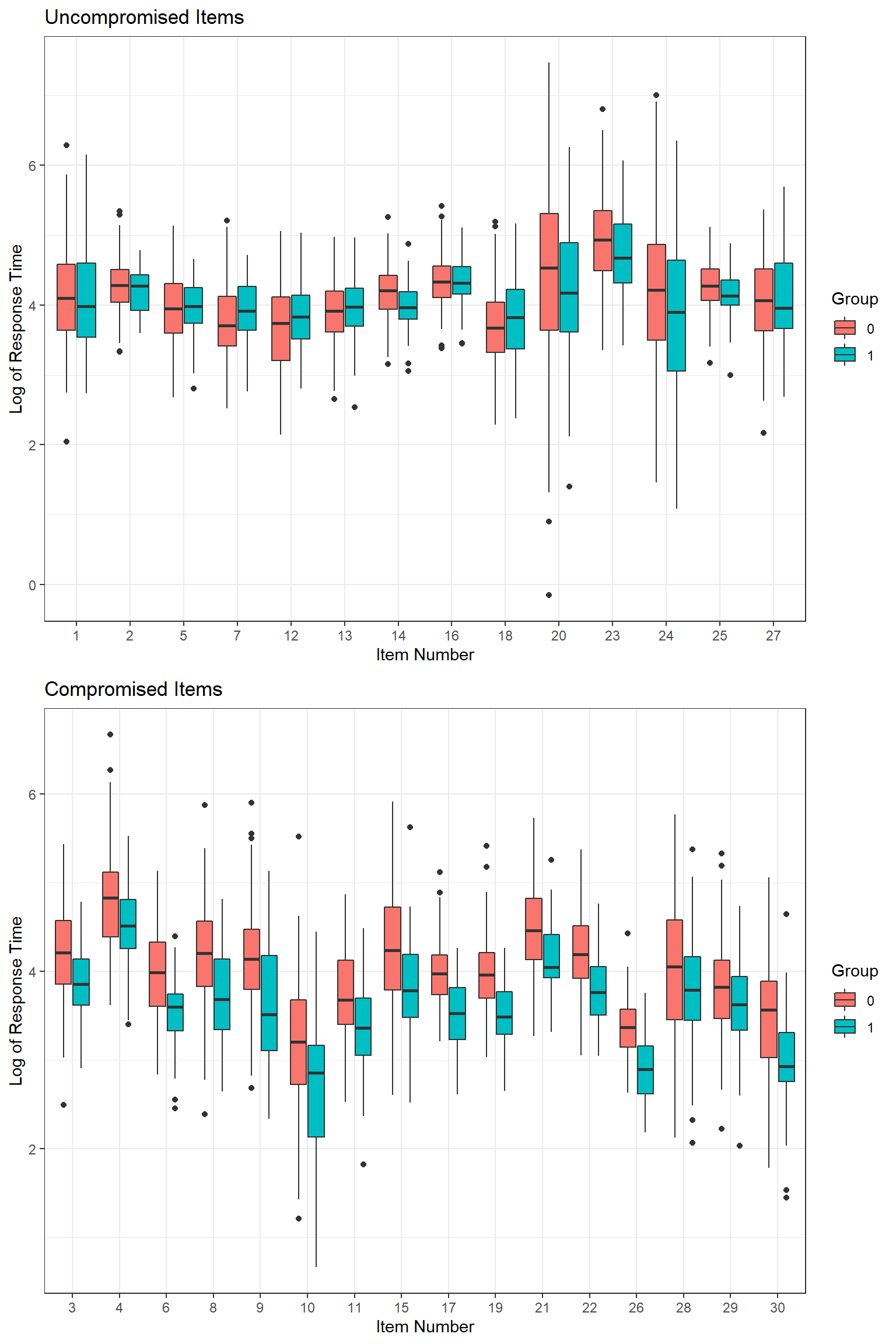### Fitting the model We first prepare a list for the input data. data_rt <- list( I = 200, J = 30, RT = log(rt[,1:30]) )  I will use the cmdstanr package to fit the model using the Stan model syntax developed before. There are four chains. There are 100 warm-up iterations followed by 500 sampling iterations for each chain. require(here) require(cmdstanr) require(rstan) mod <- cmdstan_model(here('_posts/dglnrt2/dglnrt.stan')) fit <- mod$sample(
data            = data_rt,
seed            = 1234,
chains          = 4,
parallel_chains = 4,
iter_warmup     = 100,
iter_sampling   = 500,
refresh         = 10,

fit$cmdstan_summary() stanfit <- rstan::read_stan_csv(fit$output_files())


This model took about 3 hours to run on my computer. There are so many parameters in the model. I will only focus on two for the sake of keeping this post short. These parameters are the probability of being compromised for each item and probability of each examinee having item preknowledge.

### Probability of items being compromised

As you can see the numbers below, the model predicted probability estimates perfectly separated two groups of items (disclosed and undisclosed). $$\hat{R}$$ values ranged from 0.999 to 1.002, suggesting good convergence. The probability estimates ranged from 0.12 to 0.54 with an average of 0.27 for the simulated uncompromised items, while they ranged from 0.54 to 0.86 with a mean of 0.72 for the simulated compromised items. The AUC estimate was one, indicating that the probability estimates coming out of the model did a perfect job of separating the items in these two groups. The perfect separation of two groups of items can also be seen in the density plots below. For instance, if one uses a cut-off value of 0.5 to detect whether or not an item is compromised, this model would accurately detect all compromised items while detecting only one uncompromised item as false-positive.

require(rstan)
require(psych)
require(mltools)

pC <- as.data.frame(summary(stanfit, pars = c("pC"), probs = c(0.025, 0.975))$summary) pC$trueC <- C

pC

         mean  se_mean     sd     2.5%  97.5% n_eff   Rhat trueC
pC  0.3563 0.005331 0.2633 0.012007 0.9306  2441 1.0003     0
pC  0.1932 0.004339 0.1858 0.003961 0.7395  1834 0.9986     0
pC  0.6770 0.004731 0.2252 0.172258 0.9866  2266 0.9999     1
pC  0.6837 0.004058 0.2315 0.137451 0.9871  3254 0.9988     1
pC  0.2595 0.004195 0.2037 0.010536 0.7682  2357 0.9989     0
pC  0.8133 0.003423 0.1654 0.384424 0.9958  2334 1.0002     1
pC  0.1522 0.002641 0.1467 0.003595 0.5455  3084 0.9993     0
pC  0.7864 0.004274 0.1963 0.260266 0.9928  2110 0.9999     1
pC  0.6778 0.004485 0.2187 0.170020 0.9824  2378 0.9993     1
pC 0.7160 0.004703 0.2346 0.152268 0.9923  2488 0.9991     1
pC 0.7550 0.003958 0.1945 0.275246 0.9927  2414 0.9990     1
pC 0.1787 0.002780 0.1603 0.005530 0.5975  3324 0.9988     0
pC 0.1358 0.002122 0.1230 0.005882 0.4551  3362 0.9988     0
pC 0.1870 0.003053 0.1627 0.005551 0.6030  2839 0.9985     0
pC 0.6533 0.004923 0.2526 0.104433 0.9868  2632 0.9990     1
pC 0.1161 0.001755 0.1103 0.002838 0.4098  3949 0.9986     0
pC 0.8541 0.002521 0.1309 0.508814 0.9948  2698 0.9989     1
pC 0.2686 0.003645 0.2054 0.012220 0.7619  3174 0.9996     0
pC 0.7572 0.003563 0.1796 0.342210 0.9889  2542 1.0001     1
pC 0.4645 0.005634 0.2798 0.023069 0.9560  2466 0.9997     0
pC 0.5698 0.006223 0.2741 0.048376 0.9808  1940 1.0006     1
pC 0.8236 0.002805 0.1558 0.418939 0.9957  3086 0.9998     1
pC 0.3749 0.005344 0.2551 0.020048 0.9501  2279 0.9991     0
pC 0.5375 0.005135 0.2758 0.036191 0.9696  2885 0.9998     0
pC 0.2763 0.003512 0.1531 0.035231 0.6321  1900 0.9996     0
pC 0.8555 0.002401 0.1230 0.540512 0.9951  2624 0.9994     1
pC 0.2609 0.004345 0.2118 0.006762 0.7792  2376 1.0005     0
pC 0.6474 0.005694 0.2528 0.075939 0.9825  1971 1.0024     1
pC 0.5402 0.006249 0.2645 0.058637 0.9793  1792 0.9996     1
pC 0.7360 0.003684 0.2070 0.242707 0.9902  3156 1.0006     1
describeBy(pC[,1],group=C)


Descriptive statistics by group
group: 0
vars  n mean   sd median trimmed  mad  min  max range skew
X1    1 14 0.27 0.13   0.26    0.26 0.13 0.12 0.54  0.42 0.71
kurtosis   se
X1    -0.68 0.03
----------------------------------------------------
group: 1
vars  n mean   sd median trimmed mad  min  max range  skew
X1    1 16 0.72 0.09   0.73    0.73 0.1 0.54 0.86  0.32 -0.26
kurtosis   se
X1       -1 0.02
auc_roc(preds = pC[,1],
actuals = C)

 1
plot(density(pC[C==0,1]),xlim=c(0,1),main="",ylim = c(0,4))
points(density(pC[C==1,1]),lty=2,type='l')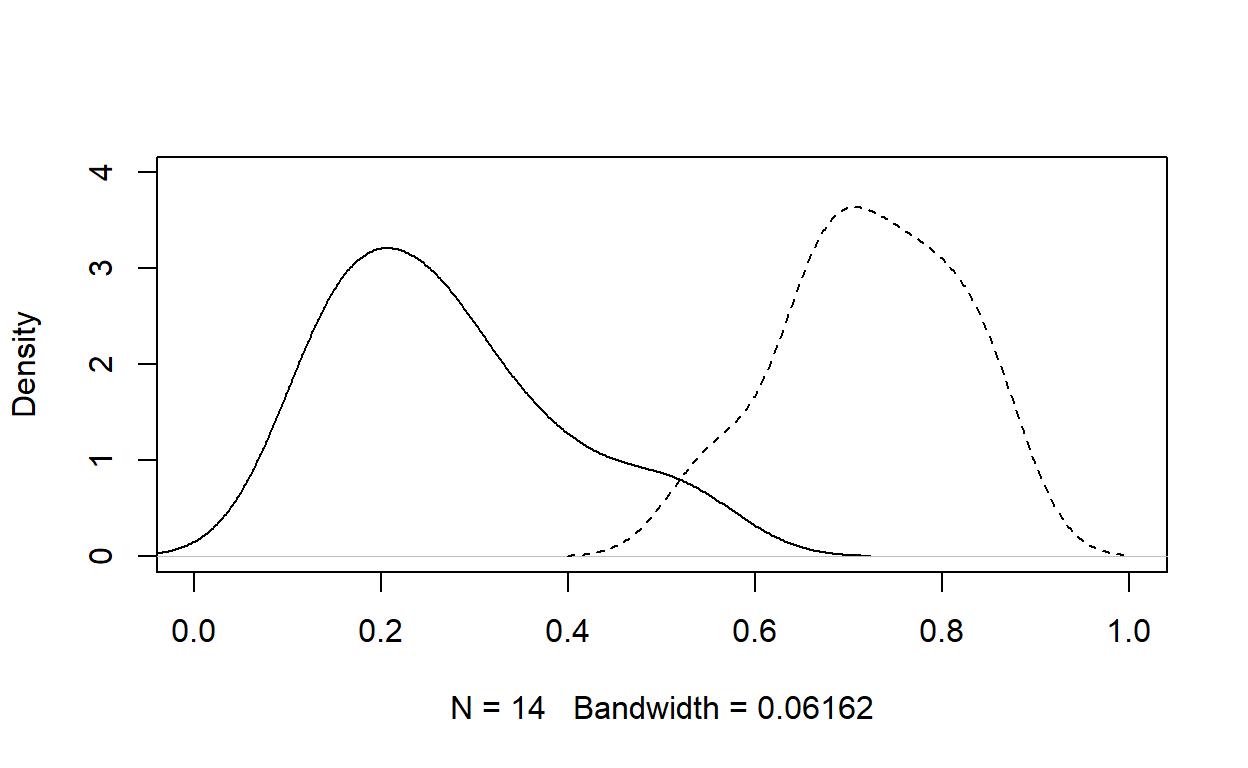table(pC$trueC,pC$mean>.5)


FALSE TRUE
0    13    1
1     0   16

### Probability of examinees having item preknowledge

The probability estimates of examinees having item preknowledge were not as perfect as the probability estimates of items being compromised. However, it still provided some promising results. $$\hat{R}$$ values ranged from 0.998 to 1.003, indicating good convergence.

The probability estimates ranged from 0.39 t0 0.64 with an average of 0.47 for the simulated examinees without item preknowledge and ranged from 0.42 to 0.84 with a mean of 0.67 for the simulated examinees with item preknowledge. The AUC estimate was about 0.95, indicating that the probability estimates coming out of the model did a reasonable job of separating the examinees with and without item preknowledge. The separation can also be seen in the density plots below.

pH       <- as.data.frame(summary(stanfit, pars = c("pH"), probs = c(0.025, 0.975))$summary) pH$trueH <- H

pH

          mean  se_mean     sd    2.5%  97.5% n_eff   Rhat trueH
pH   0.4324 0.005780 0.2824 0.01983 0.9593  2387 0.9996     0
pH   0.4136 0.005989 0.2787 0.01559 0.9511  2166 0.9999     0
pH   0.5109 0.005373 0.2874 0.02531 0.9800  2861 0.9994     0
pH   0.4206 0.005508 0.2821 0.01639 0.9614  2623 0.9988     0
pH   0.4268 0.005394 0.2904 0.01805 0.9623  2899 0.9999     0
pH   0.5801 0.006208 0.2842 0.03852 0.9822  2096 1.0004     1
pH   0.6987 0.004690 0.2344 0.12410 0.9891  2499 1.0000     1
pH   0.4221 0.005439 0.2864 0.01218 0.9622  2772 0.9990     0
pH   0.4609 0.005260 0.2880 0.02414 0.9687  2997 0.9982     0
pH  0.4394 0.005621 0.2843 0.01791 0.9597  2558 0.9995     0
pH  0.6211 0.005172 0.2663 0.08076 0.9899  2650 1.0005     1
pH  0.3967 0.005952 0.2793 0.01306 0.9511  2202 1.0008     0
pH  0.4979 0.004903 0.2857 0.02238 0.9684  3396 0.9991     0
pH  0.4384 0.005724 0.2860 0.01822 0.9726  2496 1.0003     0
pH  0.6246 0.004260 0.2510 0.10713 0.9795  3471 0.9983     1
pH  0.4910 0.005116 0.2852 0.03087 0.9741  3109 0.9996     0
pH  0.4716 0.005892 0.3009 0.01573 0.9804  2608 0.9987     0
pH  0.5877 0.005723 0.2834 0.03934 0.9857  2453 1.0004     1
pH  0.6095 0.004547 0.2557 0.08294 0.9814  3163 0.9999     1
pH  0.4515 0.005513 0.2850 0.01788 0.9746  2672 0.9993     0
pH  0.4060 0.005564 0.2795 0.02181 0.9510  2522 0.9983     0
pH  0.4139 0.005748 0.2818 0.01848 0.9596  2404 1.0014     0
pH  0.6297 0.004776 0.2633 0.07521 0.9907  3040 0.9999     0
pH  0.4744 0.005470 0.2886 0.02303 0.9710  2783 0.9997     0
pH  0.4834 0.005000 0.2819 0.02755 0.9699  3179 0.9985     0
pH  0.4323 0.005653 0.2830 0.01623 0.9650  2505 0.9984     0
pH  0.4730 0.005524 0.2868 0.02018 0.9697  2696 0.9995     0
pH  0.4979 0.004819 0.2647 0.03945 0.9614  3018 0.9999     0
pH  0.5412 0.005606 0.2734 0.04473 0.9761  2379 0.9994     1
pH  0.4560 0.005916 0.2895 0.01943 0.9708  2394 0.9997     0
pH  0.5826 0.005193 0.2714 0.04864 0.9877  2732 0.9991     0
pH  0.4468 0.005107 0.2803 0.01915 0.9721  3012 0.9989     0
pH  0.4665 0.005612 0.2961 0.01629 0.9748  2785 1.0001     0
pH  0.4796 0.005778 0.2873 0.02307 0.9687  2473 0.9989     0
pH  0.5197 0.005582 0.2828 0.03814 0.9758  2567 0.9988     0
pH  0.5581 0.005635 0.2750 0.03497 0.9746  2381 0.9989     1
pH  0.4389 0.005213 0.2841 0.02194 0.9539  2971 1.0003     0
pH  0.4187 0.005596 0.2746 0.02036 0.9573  2408 1.0004     0
pH  0.5116 0.005338 0.2863 0.03419 0.9801  2876 0.9989     0
....
plot(density(pH[H==0,1]),xlim=c(0,1),main="")
points(density(pH[H==1,1]),type='l',lty=2)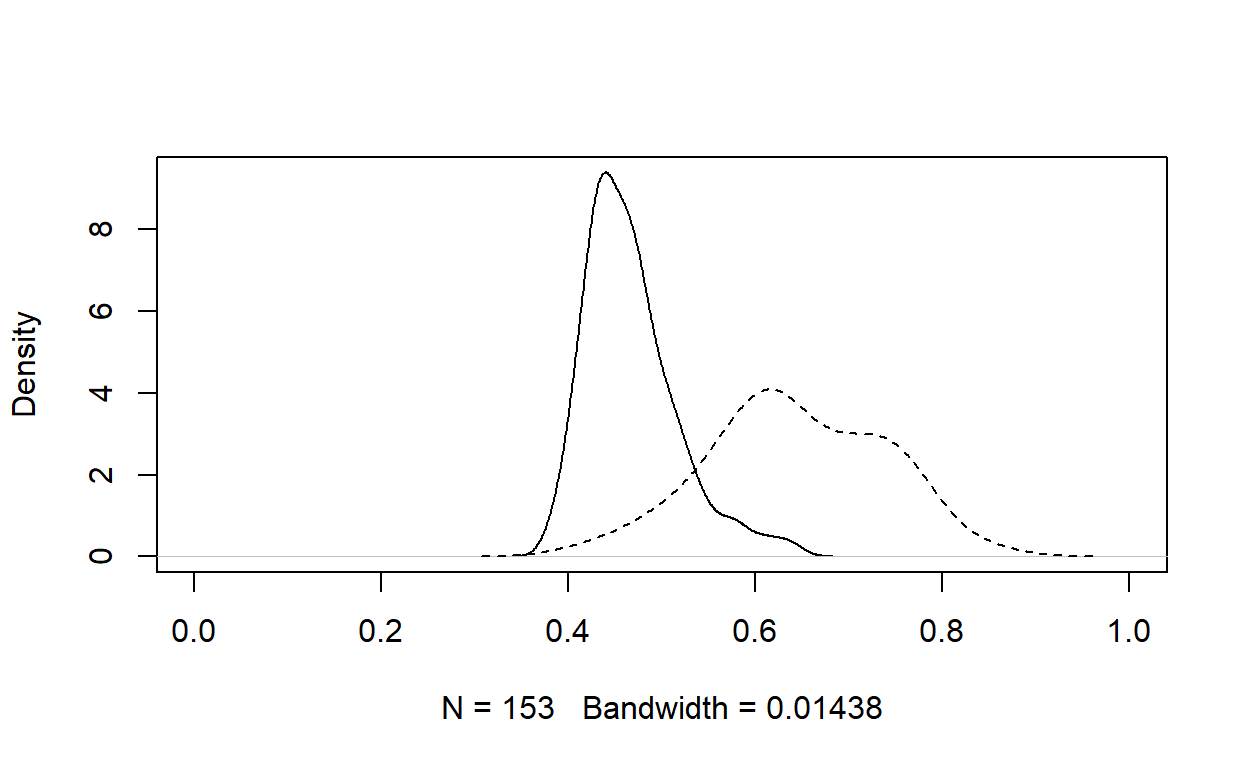describeBy(pH[,1],group=H)


Descriptive statistics by group
group: 0
vars   n mean   sd median trimmed  mad  min  max range skew
X1    1 153 0.47 0.05   0.46    0.46 0.04 0.39 0.64  0.25 1.09
kurtosis se
X1      1.3  0
----------------------------------------------------
group: 1
vars  n mean   sd median trimmed mad  min  max range  skew
X1    1 47 0.64 0.09   0.63    0.65 0.1 0.42 0.84  0.42 -0.11
kurtosis   se
X1    -0.54 0.01
auc_roc(preds = pH[,1],
actuals =H)

 0.9492

For instance, if one uses a cut-off value of 0.6 to detect whether or not an examinee has item preknowledge, we would get the following confusion matrix, yielding a false-positive rate of 0.026, true-positive rate of 0.68, and precision of 0.89.

table(pH$trueH,pH$mean>.6)


FALSE TRUE
0   149    4
1    15   32

## Real Data Example

In this dataset, there are 93 examinees and 25 items. Below is the first three rows and 5 columns (not allowed to publicize this dataset). The first two columns are variables for a unique identification number and a group membership. The last 25 items include the observed response time for 25 items in the test.

  ID COND  Q1RT  Q2RT   Q3RT
1  1    2 35.59 15.05  68.66
2  2    1 70.30 67.82 126.90
3  3    3 50.45 34.71 299.22
 93 27

1  2  3
33 30 30 

One group (Group 1) was a control group, and they responded to all 25 items without any preknowledge. The second and third groups were experimental groups. Group 2 was allowed to study 12 items (even-numbered items) without the correct key, while Group 3 was allowed to study the same 12 items with the correct key before taking the test. The plots below show the distribution of log response time within each group for odd-numbered items and even-numbered items. While there is not much difference among the groups for the undisclosed odd-numbered items, the experimental effect reveals itself with shorter response times in disclosed even-numbered items for the examinees in Group 2 and 3. So, in theory, the model should successfully separate these three groups of examinees by assigning a higher probability of item preknowledge for examinees in Group 2 and Group 3. Also, the model should successfully separate two groups of items by assigning a higher probability of being compromised for the even-numbered items.

### Data Check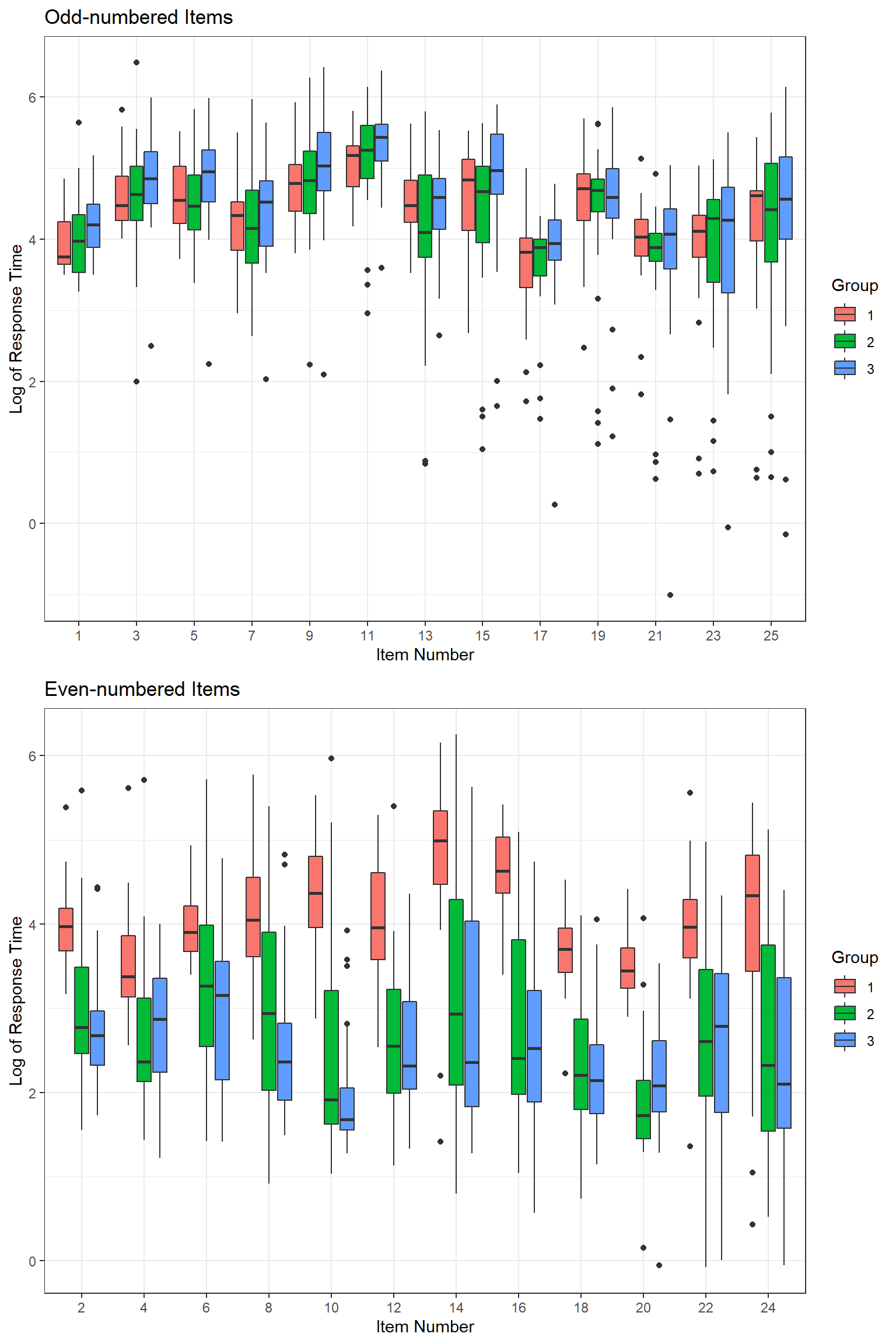### Model Fitting

We first prepare a list for the input data.

data_rt <- list(
I               = 93,
J               = 25,
RT              = log(d.sub[,3:27])
)


I will use the cmdstanr package to fit the model using the Stan model syntax developed before. There are four chains. There are 100 warm-up iterations followed by 500 sampling iterations for each chain.

require(cmdstanr)

mod <- cmdstan_model(here('_posts/dglnrt2/dglnrt.stan'))

fit <- mod$sample( data = data_rt, seed = 1234, chains = 4, parallel_chains = 4, iter_warmup = 100, iter_sampling = 500, refresh = 10, adapt_delta = 0.99) fit$cmdstan_summary()

stanfit <- rstan::read_stan_csv(fit$output_files())  It took about 35 minutes to run on my computer. ### Probability of items being compromised As you can see the numbers below, the model predicted probability estimates perfectly separated two groups of items (disclosed and undisclosed). $$\hat{R}$$ values ranged from 1 to 1.22. I should probably increase the number of warm-up and sampling iterations and re-run to get better convergence, but I think these are good enough for the sake of this demo. The probability estimates ranged from 0.02 t0 0.39 with an average of 0.14 for the undisclosed items, while they ranged from 0.81 to 0.98 with a mean of 0.92 for the disclosed items. The AUC estimate was one indicating a perfect separation of the disclosed and undisclosed items. The perfect separation of two groups of items can also be seen in the density plots below. For instance, if one uses a cut-off value of 0.5 to detect whether or not an item is compromised, this model would perfectly recover the disclosed items in the experimental setting. pC <- as.data.frame(summary(stanfit, pars = c("pC"), probs = c(0.025, 0.975))$summary)
pC$trueC <- c(rep(c(0,1),12),0) pC   mean se_mean sd 2.5% 97.5% n_eff Rhat trueC pC 0.02279 0.0003900 0.02248 0.0004506 0.0837 3324 0.9989 0 pC 0.89192 0.0013721 0.06822 0.7318409 0.9901 2472 0.9998 1 pC 0.06429 0.0007507 0.03883 0.0105791 0.1536 2676 0.9986 0 pC 0.81685 0.0021437 0.10149 0.6056597 0.9846 2241 0.9993 1 pC 0.04235 0.0005289 0.03252 0.0022409 0.1231 3781 0.9993 0 pC 0.81485 0.0019671 0.10300 0.5808236 0.9804 2742 1.0001 1 pC 0.04267 0.0006814 0.03823 0.0011927 0.1470 3147 0.9986 0 pC 0.90350 0.0012449 0.06826 0.7435097 0.9945 3007 0.9992 1 pC 0.07053 0.0008697 0.04076 0.0137320 0.1691 2196 0.9988 0 pC 0.96293 0.0005276 0.03304 0.8749595 0.9990 3922 0.9986 1 pC 0.06193 0.0006584 0.03901 0.0066121 0.1538 3511 0.9991 0 pC 0.96692 0.0005443 0.03160 0.8823371 0.9991 3370 0.9987 1 pC 0.12841 0.0013142 0.05894 0.0366359 0.2590 2012 1.0007 0 pC 0.90235 0.0012686 0.06766 0.7481475 0.9943 2845 0.9982 1 pC 0.17636 0.0015006 0.06346 0.0702638 0.3134 1788 0.9993 0 pC 0.95090 0.0007565 0.04326 0.8438114 0.9982 3270 0.9993 1 pC 0.14062 0.0012718 0.04873 0.0619892 0.2493 1468 1.0002 0 pC 0.97221 0.0004352 0.02676 0.9038320 0.9993 3782 0.9989 1 pC 0.17644 0.0013468 0.05956 0.0753981 0.3037 1955 1.0007 0 pC 0.97648 0.0004188 0.02381 0.9119659 0.9995 3234 0.9990 1 pC 0.18806 0.0014627 0.06141 0.0847824 0.3196 1763 0.9989 0 pC 0.94778 0.0007269 0.04725 0.8258265 0.9985 4225 0.9987 1 pC 0.38546 0.0018617 0.08426 0.2317135 0.5528 2048 0.9987 0 pC 0.95307 0.0007975 0.04418 0.8357623 0.9990 3068 0.9983 1 pC 0.30796 0.0018838 0.08074 0.1618400 0.4814 1837 1.0008 0 describeBy(pC$mean,group=pC$trueC)   Descriptive statistics by group group: 0 vars n mean sd median trimmed mad min max range skew kurtosis X1 1 13 0.14 0.11 0.13 0.13 0.1 0.02 0.39 0.36 0.91 -0.31 se X1 0.03 ---------------------------------------------------- group: 1 vars n mean sd median trimmed mad min max range skew X1 1 12 0.92 0.06 0.95 0.93 0.04 0.81 0.98 0.16 -0.84 kurtosis se X1 -0.83 0.02 auc_roc(preds = pC$mean,
actuals = pC$trueC)   1 plot(density(pC[pC$trueC==0,1]),xlim=c(0,1),main="",ylim = c(0,10))
points(density(pC[pC$trueC==1,1]),lty=2,type='l')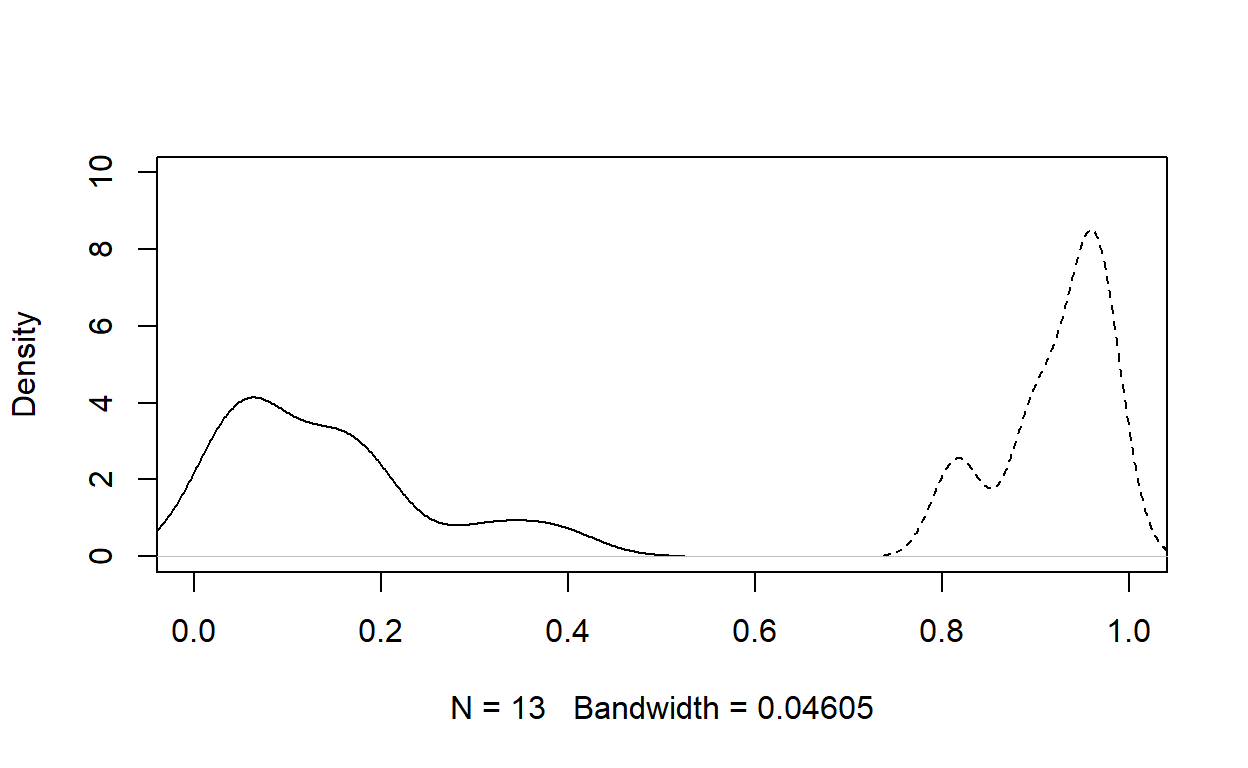table(pC$trueC,pC$mean>.5)   FALSE TRUE 0 13 0 1 0 12 ### Probability of examinees having item preknowledge The probability estimates of examinees having item preknowledge were almost as good as the probability estimates of items being compromised. $$\hat{R}$$ values ranged from 0.983 to 1.006. The probability estimates ranged from 0.19 t0 0.46 with an average of 0.32 for the examinees in the control group (no preknowledge), while they ranged from 0.30 to 0.94 with a mean of 0.72 for the second group (having preknowledge without the correct responses) and ranged from 0.50 to 0.95 with a mean of 0.82 for the third group (having preknowledge with the correct responses). The AUC estimate was 0.98, indicating a good degree of separation among the examinees in these three groups. The separation can also be seen in the density plots below. pH <- as.data.frame(summary(stanfit, pars = c("pH"), probs = c(0.025, 0.975))$summary)
pH$trueH <- ifelse(d.sub$COND==1,0,1)
pH

         mean   se_mean      sd     2.5%  97.5%  n_eff   Rhat trueH
pH  0.3739 0.0033378 0.16536 0.122342 0.7767 2454.5 1.0000     1
pH  0.4207 0.0070813 0.28521 0.011273 0.9436 1622.3 1.0003     0
pH  0.7659 0.0025472 0.16281 0.403952 0.9892 4085.5 0.9992     1
pH  0.8687 0.0022007 0.12117 0.543230 0.9974 3031.5 0.9990     1
pH  0.5147 0.0029313 0.14882 0.238189 0.8025 2577.7 1.0005     1
pH  0.3002 0.0080551 0.26823 0.006125 0.9330 1108.8 0.9994     0
pH  0.9108 0.0013505 0.07872 0.710695 0.9979 3397.7 1.0007     1
pH  0.3093 0.0074335 0.27576 0.006109 0.9203 1376.2 1.0002     0
pH  0.7587 0.0025103 0.15675 0.407047 0.9869 3899.3 0.9987     1
pH 0.4637 0.0083978 0.29984 0.022981 0.9781 1274.8 1.0015     0
pH 0.9288 0.0010362 0.06615 0.756134 0.9975 4075.2 0.9990     1
pH 0.3201 0.0071752 0.26811 0.007590 0.9103 1396.2 0.9997     0
pH 0.9138 0.0012575 0.07982 0.706961 0.9970 4028.7 0.9998     1
pH 0.7041 0.0025965 0.17764 0.337529 0.9813 4680.7 0.9988     1
pH 0.8360 0.0021308 0.13367 0.484906 0.9942 3935.3 0.9986     1
pH 0.2553 0.0021182 0.10702 0.082439 0.4883 2552.8 0.9985     0
pH 0.3125 0.0080216 0.27730 0.006544 0.9434 1195.0 1.0023     0
pH 0.7939 0.0020365 0.12933 0.503429 0.9765 4033.2 0.9984     1
pH 0.8375 0.0017937 0.11516 0.564244 0.9921 4121.6 0.9995     1
pH 0.3023 0.0025411 0.11439 0.102210 0.5504 2026.5 1.0002     0
pH 0.8513 0.0017211 0.11240 0.579003 0.9932 4264.8 0.9984     1
pH 0.8032 0.0034052 0.17463 0.353354 0.9933 2630.1 0.9994     1
pH 0.4993 0.0028453 0.17052 0.182437 0.8394 3591.6 0.9983     1
pH 0.3269 0.0089522 0.29332 0.006415 0.9582 1073.5 1.0012     0
pH 0.5905 0.0029961 0.17361 0.261305 0.9003 3357.6 0.9986     1
pH 0.6889 0.0023612 0.14412 0.387882 0.9471 3725.7 1.0004     1
pH 0.3241 0.0072829 0.27493 0.006545 0.9241 1425.1 0.9999     0
pH 0.9079 0.0013019 0.08094 0.699641 0.9966 3865.1 0.9994     1
pH 0.2670 0.0091847 0.27534 0.004448 0.9344  898.7 1.0027     0
pH 0.3494 0.0087796 0.29115 0.008960 0.9549 1099.8 1.0059     0
pH 0.7718 0.0026901 0.16225 0.402562 0.9865 3637.5 0.9987     1
pH 0.9460 0.0009635 0.05386 0.798829 0.9988 3124.2 0.9999     1
pH 0.8285 0.0023823 0.13438 0.498653 0.9934 3181.8 0.9995     1
pH 0.8664 0.0019269 0.11983 0.559261 0.9957 3866.9 0.9994     1
pH 0.2598 0.0078278 0.23986 0.016484 0.8936  939.0 1.0025     0
pH 0.6521 0.0026381 0.11940 0.411404 0.8725 2048.6 0.9997     1
pH 0.3112 0.0088663 0.27708 0.006535 0.9422  976.6 1.0007     0
pH 0.6841 0.0026520 0.16076 0.355268 0.9535 3674.6 0.9992     1
pH 0.7209 0.0024327 0.14626 0.405216 0.9577 3614.7 0.9989     1
....
plot(density(pH[which(d.sub$COND==1),1]),xlim=c(0,1),main="") points(density(pH[which(d.sub$COND==2),1]),type='l',lty=2)
points(density(pH[which(d.sub$COND==3),1]),type='l',lty=3)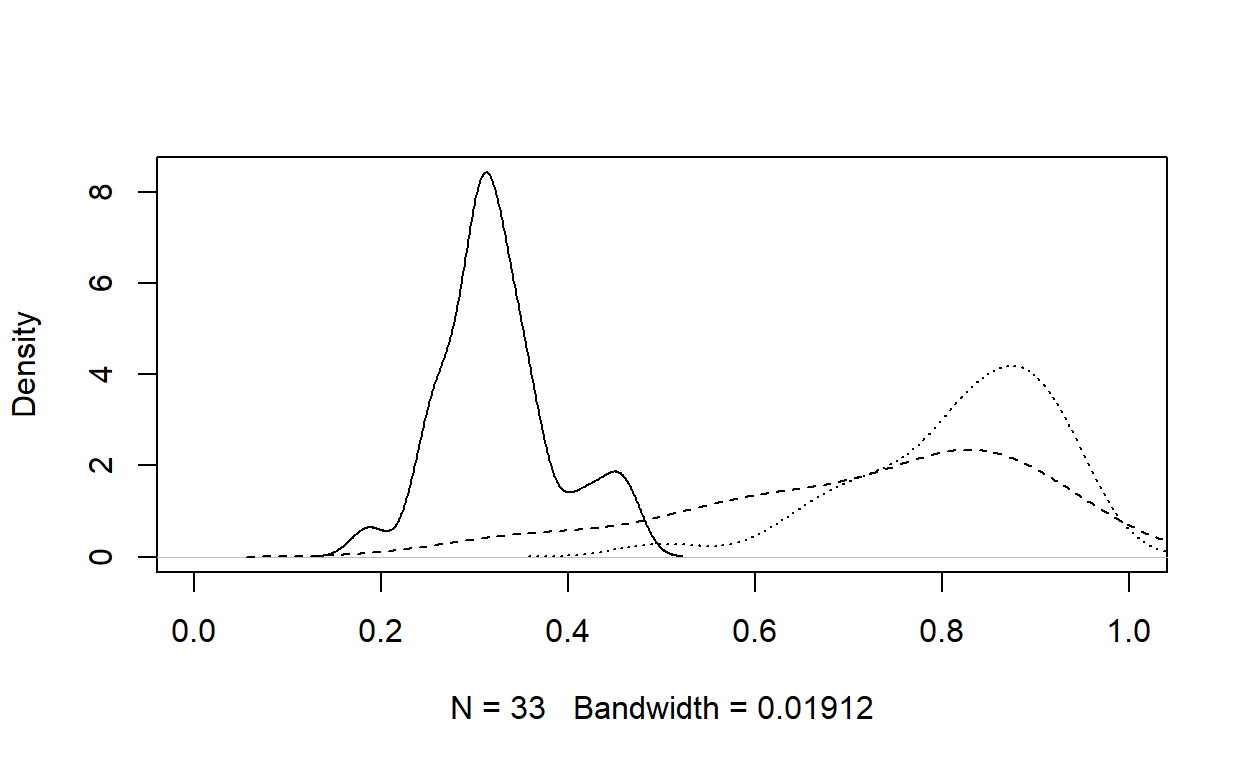describeBy(pH$mean,group=d.sub$COND)   Descriptive statistics by group group: 1 vars n mean sd median trimmed mad min max range skew X1 1 33 0.32 0.06 0.31 0.32 0.05 0.19 0.46 0.28 0.46 kurtosis se X1 0.07 0.01 ---------------------------------------------------- group: 2 vars n mean sd median trimmed mad min max range skew X1 1 30 0.72 0.18 0.78 0.73 0.17 0.3 0.94 0.64 -0.72 kurtosis se X1 -0.52 0.03 ---------------------------------------------------- group: 3 vars n mean sd median trimmed mad min max range skew kurtosis X1 1 30 0.82 0.1 0.84 0.83 0.11 0.5 0.95 0.45 -1.1 0.79 se X1 0.02 auc_roc(preds = pH$mean,
actuals = pH$trueH)   0.9828 For instance, if one uses a cut-off value of 0.5 to detect whether or not an examinee has item preknowledge, we would get the following confusion matrix, yielding a false-positive rate of 0, true-positive rate of 0.92, and precision of 1. table(pH$trueH,pH\$mean>.5)


FALSE TRUE
0    33    0
1     5   55

## Some Considerations

I think we have a decent model that may potentially work in practice. The most important thing about this model is that it does not assume that the compromised items are known. The model returns a probability of an item being compromised and a probability of an examinee having item preknowledge. Below are some considerations about this model as I work on improving it:

• The model can easily be generalized to incorporate actual item response data (0: correct, 1:incorrect), as a modified version of van der Linden’s Hierarchical IRT model. The model performance would potentially be better by incorporating information from both item responses and response times (it is almost done, will be posted soon!).

• The model is flexible enough to incorporate partial information. Suppose that you know certain items were compromised (e.g., through web monitoring) and certain examinees had item preknowledge (e.g., confession). This information can be provided to the model by fixing the relevant values to 1 in the transformed parameters block.

• The promising results in this post using the experimental data from Toton and Maynes (2019) are probably overestimating how this model would perform in a real setting. We know that the effect size of item preknowledge on response times in this dataset is massive, yielding 70%-80% reductions in response time. Similarly, the item preknowledge effect in the simulated data example yields on average about 35% reduction in response time. So, there is more than enough signal in both real and simulated datasets for the model to work in an ideal condition. The effect size in natural settings is probably much smaller (e.g., a 10%-20% reduction in response times). Also, there will be more noise with different contributing factors to response times (e.g., rapid guessing). In such conditions, it will be more difficult for the model to detect items and examinees.

• Both the real and simulated datasets used for demonstration provides an ideal setting in which the same examinees had access to the same set of items, and they were provided the correct key. Any deviation from this perfect scenario may deteriorate the model performance, such as smaller groups having access to a different subset of items or the disclosed keys are flawed.

### Citation

Zopluoglu (2021, June 24). Cengiz Zopluoglu: Simultaneous Detection of Compromised Items and Examinees with Item Preknowledge using Response Time Information. Retrieved from https://github.com/czopluoglu/website/tree/master/docs/posts/dglnrt2/
@misc{zopluoglu2021simultaneous,
}